# Pythagorean theorem - examples - page 6

1. HeightIs right that in any right triangle height is less or equal half of the hypotenuse?
2. Hexagonal pyramidCalculate the volume and the surface of a regular hexagonal pyramid with a base edge length 3 cm and a height 5 cm.
3. Right triangleCalculate the missing side b and interior angles, perimeter and area of ​​a right triangle if a=10 cm and hypotenuse c = 16 cm.
4. Two forcesTwo forces with magnitudes of 25 and 30 pounds act on an object at angles of 10° and 100° respectively. Find the direction and magnitude of the resultant force. Round to two decimal places in all intermediate steps and in your final answer.
5. Track arc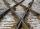Two straight tracks is in an angle 74°. They will join with circular arc with radius r=1127 m. How long will be arc connecting these lines (L)? How far is the center point of arc from track crossings (x)?
6. Airplane navigationAn airplane leaves an airport and flies due west 120 miles and then 150 miles in the direction S 44.1°W. How far is the plane from the airport (round to the nearest mile)?
7. Perimeter and legsDetermine the perimeter of a right triangle if the length of one leg is 75% length of the second leg and its content area is 24 cm2.
8. Prism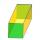The lenght, width and height of a right prism are 6, 17 and 10 respectively. What is the lenght of the longest segment whose endpoints are vertices of the prism?
9. Rotating cone IICalculate area of surface of rotating cone with base radius r=19 cm and height h=9 cm.
10. V-belt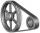Calculate the length of the belt on pulleys with diameters of 105 mm and 393 mm at shaft distance 697 mm.
11. Two ballsTwo balls, one 8cm in radius and the other 6cm in radius, are placed in a cylindrical plastic container 10cm in radius. Find the volume of water necessary to cover them.
12. ArmCalculate the length of the arm r of isosceles triangle ABC, with base |AB| = 18 cm and a height v=17 cm.
13. Cone and the ratioRotational cone has a height 23 cm and the ratio of the base surface to lateral surface is 7: 9. Calculate a surface of the base and the lateral surface.
14. Q-Exam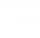If tg α = 0.9, Calculating sin α, cos α, cotg α .
15. EQL triangle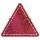Calculate inradius and circumradius of equilateral triangle with side a=77 cm.
16. VectorCalculate length of the vector v⃗ = (9.75, 6.75, -6.5, -3.75, 2).
17. Equilateral triangleCalculate the side of an equilateral triangle, if its area is 892 mm2.
18. Euklid4Legs of a right triangle have dimensions 244 m and 246 m. Calculate the length of the hypotenuse and the height of this right triangle.
19. Hexagonal prismThe base of the prism is a regular hexagon consisting of six triangles with side a = 12 cm and height va = 10.4 cm. The prism height is 5 cm. Calculate the volume and surface of the prism!
20. Sphere and coneWithin the sphere of radius G = 33 cm inscribe cone with largest volume. What is that volume and what are the dimensions of the cone?

Do you have an interesting mathematical example that you can't solve it? Enter it, and we can try to solve it.

To this e-mail address, we will reply solution; solved examples are also published here. Please enter e-mail correctly and check whether you don't have a full mailbox.

Pythagorean theorem is the base for the right triangle calculator.